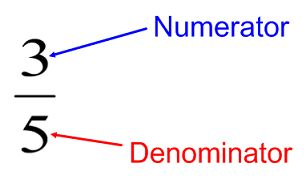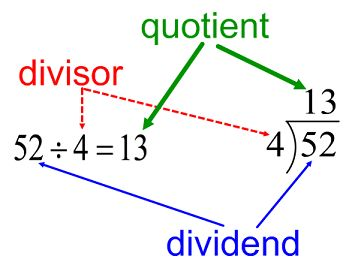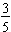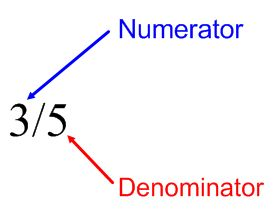# Background Knowledge

## Terms

• Numerator is the top number of the fraction.
• Denominator is the bottom number of the fraction.
• In division, the number you dividing by is the divisor. The answer is the quotient. (The beginning number is call the "dividend," but this term isn't used as often as the other terms.Fractions can be written with a horizontal line or the "slash," which is common with computers. For example, three-fifths,, can be written 3/5.